Monday, October 31, 2011

Any numerical computing environment on Java platform?

Recently Tomas Petricek and I have co-written a book chapter, Numerical Computing in F#. It is a free online book chapter for his Manning book: Real World Functional Programming. This book chapter is a survey on numerical computing for .Net languages, specially for F#. I will write another article on this book chapter and present some materials that were cut down from final version on MSDN.

But today, I am looking for things on our competitor’s side -- numerical computing environments for Java platform. I am just wondering what Java guys are doing for numerical computing.

Looking at the mature numerical systems such as Matlab, Mathematica and S-plus/R, we can summarize three general elements of such a system:

1. Math library. Math library is a must for serious numerical computing. It is hard to build well-tested numerical procedures. This kind of low level stuff, e.g. linear algebra routines and FFT, is usually available as a reusable software component.

2. Interactive/Console. The Read-eval-print loop (REPL) is very important for exploratory analysis because it gives a quick feedback on some small fragments of code.

3. Plot/chart library. A plot explains what’s going on clearly and quickly!

I did some web search and found quite a few blog articles; I want to mention articles by Mikio L. Braun. He also maintains his jblas library, which is a JNI wrapper to ATLAS, the highly-optimized BLAS library.

I am aware Incanter, which is a Clojure project aiming to be R in Java platform. But I don't think rewriting basic things, e.g. PCA and linear regression, for Java Platform is a good idea since a stable implementation for these algorithms usually take years because there is just too much details in numerical algorithms.

This time, I found a new library, ScalaLab, which is a on-going project for numerical computing in Scala.

Let’s first talk the library a little bit. For matrix and linear algebra, there are also two quite stable Java libraries: colt and JAMA. The linear algebra functionality of the two libraries should be intact. But the performance is quite bad compared to native code, even several times slower to pure .Net code. [The comparison to .Net code is my personal experience. ] But one should not care too much about performance as the bottleneck of a numerical project differs from projects to projects. A working system is the most important thing, various methods could help to improve the performance. Btw, the performance difference is usually not noticeable when we work on small or sample datasets. I use R quite often recently, the matrix library associated with its Windows version is from Netlib, a normal native performance one. I didn’t have any performance complain over months.

For REPL, basically it is a language issue. After reading through online, I think I will like something built on Scala, such as the ScalaLab environment. Among the mature/quite-mature JVM languages, Scala is most similar to F#. The advantage of Scala to F# is that Scala is a component language, which means Scala has better OO features for library designers and software architects. However, on the small/low level of programming (e.g. a small function), F# feels much more functional and pure than Scala. This, I think, is partially because F# “IS” an ML, while Scala borrows some syntax from Java and C#. The other reason is that OO is at the heart of Scala – everything is an object. Scala’s OO model is greatly influenced by Smalltalk’s. I haven’t learned any Smalltalk. But after some learning in Scala, I can appreciate why Alan Kay, the inventor of Smalltalk, says, “Actually I made up the term "object-oriented", and I can tell you I did not have C++ in mind. “. So even functional programming in Scala is OO-emulated, while F# has a functional base first and then the implementation using .Net is transparent to its syntax. Ok… the above comparison is just off the topic. Anyway, Scala can serve as a good static script language with a REPL.

For the plotting library, JFreeChart seems to be the standard. It is also the plotting library used in Incanter [For this part, I think Incanter really does a great job!]. I am not sure whether there are some advanced or commercial plotting libraries for Java. But I know that .Net has tons of that! Anyway, JFreeChart is ok for daily use.

The last thing, which I haven’t listed above, is parallel computing! Java platform, or more specific, the Java Virtual Machine, proves to be one of the most stable multithreaded systems ever built. For example, Hadoop is written in Java. Although people in Google said that Hadoop is way slow compared to their C++ MapReduce. A lot of big companies are using it. Two months ago, I read the following nice paper by Martin Odersky and Kunle Olukotun:

Language Virtualization for Heterogeneous Parallel Computing

It is just published and already has 28 citations on Google Scholar. The idea is to build a DSL, which serves a middle ware, to write high level programs, e.g. equations involving some matrix operations. Underlying the DSL, there are complicated optimizations going on to transform it and make it parallel using heterogeneous technologies, e.g. GPU and multi-core. So the programmer does not need to know anything about parallel computing and his program is executed in parallel utilizing the multi-cores! The DSL is an internal one built in Scala, another example showing the great expressiveness of Scala. Since such idea is not new, the novel part of the paper is to demonstrate how Scala perfectly fits the requirements of such a paradigm.

I don’t quite have a conclusion for this post. Just some random thoughts on numerical computing in Java and an advertisement for my articles on MSDN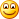Wednesday, September 14, 2011

A functional red black tree with dynamic order statistics in F#

Yin Zhu

14 September 2011

Binary search trees play an extremely important role in computer science. They were hot research topics back to 1970s and early 1980s. While the plain binary search tree is of little usage, balanced search trees are now being used everywhere. For example, most C++ STL implementations use Red Black Tree for its set and map containers. The F# Core library uses a functional version of AVL tree for Set and Map, which are both persistent/functional containers.

Special binary search trees also have other functionalities other than implementing standard set or map containers. For example, splay tree has an efficient split operation – splitting a dynamic set into two in O(log n) time: one is less than the given key; the other is greater than or equal to the given key. This operation could be used to optimize network flow algorithms. Details could be found in Tarjan’s classical (also tiny) data structures book published in 1983.

In this post, I’d like to implement the classical Red Black Tree in F#. I searched on the internet and found that there were some Red Black Tree code snippets in F#, but none of them are complete. Some only implemented the insert operation, which is relatively easy. Some are incorrect.

My main purpose for implementing the Red Black Tree in F# is to test whether I am still good at implementing complex data structures (I implemented quite a few classical data structures and algorithms during my early undergraduates for ACM/ICPC competitions). But there are new things: 1) the implementation language is F# rather than C++, 2) the implementation should be functional rather than imperative, and 3) the interface design should be general, and allows code reuse.

One function I always miss from standard set containers is dynamic order statistics – finding the ith smallest element in a dynamic set. The query time should be in O(log n). To accomplish this, we need add a size field to the internal tree node. Adding extra fields into a data structure is called augmenting data structures (Chapter 14, CLRS). Augmenting data structures is useful when a function is not built in a classical data structure but can be added by genuinely modifying the classical data structure. It is a very important skill when solving algorithmic competition problems.

I divided this article into several sections. I will first introduce the basics of red black trees, and then we move the insertion part, and order statistics, and then the hardest part – deletion. I have a section describing the testing procedures that ensure the correctness of the implementation. At last, I will leave the specifics of Red Black Trees, and cover the tree traversal in general and show some more functional stuff: continuation and a continuation Monad.

Red Black Tree Basics

A Red Black Tree is a binary search tree with the following extra properties:

1. The root is black and the leaf nodes are black (note: leaf nodes do not contain keys, only internal nodes contain keys).

2. A red node’s two children must be black.

3. For any subtree rooted at x, all the simple paths down to the leaf nodes contain the same number of black nodes.

With the above properties, the Red Black tree is able to perform queries such as search, insert and delete all in O(log n) time.

The F# definition of the tree is given below:

type Color =
Red | Black | DoubleBlack

type RBTree<'a when 'a:comparison> =
| Node of Color * 'a * int * RBTree<'a> * RBTree<'a>
| Leaf of Color

Notice that a node could be Red or Black. For black, there are two cases Black and DoubleBlack. The DoubleBlack color is used only when we are dealing with deletion, and in a valid Red Black tree, there would be of no DoubleBlack node. I will explain DoubleBlack in the delete section.

A Red Black Tree has two kinds of nodes: internal nodes storing keys and dummy leaf nodes. The internal node has a color field, the key filed, the size of the subtree and left and right children. The leaf node has two possible colors: Black or DoubleBlack.

Let’s write two simple recursive functions working on the tree structure to get us warmed up:

let rec contains x = function
| Leaf _ -> false
| Node (_, y, _, a, b) ->
if
x = y then true
elif
x < y then contains x a
else contains x b

let rec depth = function
| Leaf _ -> 0
| Node (_, _, _, a, b) ->
1 + max (depth a) (depth b)

The first is to test whether a key x is in the tree or not. The second is to calculate the depth of a tree.

Insert

For insertion, there is a known trick found by Chris Okasaki in this paper (Red-black trees in a functional setting). Okasaki is also the author of the famous book, Purely Functional Data Structures.

The idea of insertion is: always insert a Red node at some leaf node so that property 3 holds, then fix property 2 up to the root. There are four cases where property 2 could break. For all the four cases, the grand parent is always black, and there will be two red nodes right in the below. Please see the picture on page 3 of the paper.

Then the buttom-up fixing goes up the root. The root may be changed into Red. To hold property 1, simply change the color of the root to Black.

let insert x (t: RBTree<'a>) =
let balance = function
| Black, z, size, Node (Red, y, _, Node (Red, x, _, a, b), c), d
| Black, z, size, Node (Red, x, _, a, Node (Red, y, _, b, c)), d
| Black, x, size, a, Node (Red, z, _, Node (Red, y, _, b, c), d)
| Black, x, size, a, Node (Red, y, _, b, Node (Red, z, _, c, d))->
Node (Red, y, size, Node (Black, x, (getSize a b)+1, a, b), Node (Black, z, (getSize c d)+1, c, d))
| color, b, _, c, d -> // grandparent is red, does not change
Node (color, b, (getSize c d)+1, c, d)

let rec ins = function
| Leaf _ ->
Node (Red, x, 1, Leaf(Black), Leaf(Black))
| Node (color, y, size, a, b) as s ->
if
x < y then balance(color, y, (getSize a b)+2, ins a, b)
elif x > y then balance(color, y, (getSize a b)+2, a, ins b)
else s

match ins t with
| Node (Red, y, size, a, b)->
Node (Black, y, size, a, b)
| t -> t

Order statistics

Let’s have some rest before going to the monstrous delete operation. To get the nth smallest key in the tree, we can add a size field to each node, which is the size of the subtree rooted at the node. Thus the size of Node(_, _, _, left, right) is defined as

size = left.size + right.size + 1

and, as a boundary condition, a leaf node has size 0.

With this size field, the nth function is given below:

let nth n t =
let rec nth' n = function
| Leaf _->
failwith "can't find the nth member"
| Node (_, v, size, l, r)->
let
lsize=getSize l (Leaf(Black))
if lsize+1=n then
v
elif lsize+1>n then
nth' n l
else
nth' (n-lsize-1) r
if n >= size t || n < 0 then
failwith "nth out of range"
else
nth' (n+1) t

Delete

The idea of delete is simple (if we don’t care about the three properties of Red Black Trees): if the node x to be deleted has less than two children, then simply delete it, and lift its child (if any) to its position; if the node has two children, that means there must a node that is just bigger than x in its right child, replace x with that value, now the problem reduces to delete the smallest node in x’s right child.

First let’s write the function to get the smallest value in a tree:

let rec min = function
| Leaf _->
None
| Node (_, value, _, Leaf(Black), _)->
Some(value)
| Node (_, _, _, l, r)->
min l

Then we continue to code the delete logic:

let rec del value = function
| Leaf(color) ->
failwith "RBT delete failed because x is not in the tree"
| Node (color, y, _, a, b) as s ->
if
value < y then balance(color, y, 0, del value a, b)
elif value > y then balance(color, y, 0, a, del value b)
else
match
(a, b) with
| (Leaf(Black), Leaf(Black)) -> // without non-leaf children
Leaf(Black++color)
| (Leaf(Black), Node (nc, nv, size, nl, nr))
| (Node (nc, nv, size, nl, nr), Leaf(Black)) -> // with a single child
Node (color++nc, nv, size, nl, nr)
| (l, r) ->
//find the successor (smallest element in a right child),
//replace the key with the successor's
//and delete relevant node
match (min r) with
| Some(v) ->
balance(color, v, 0, l, del v r)
| None ->
failwith "impossible: can't find the successor"

The hard part is how to balance the tree after the deletion of the node. If we delete a black node, then Property 3 cannot hold anymore. So we must make rotations and do some rearrangements on colors to make Property 3 to hold again.

Think this problem in another way: after the deletion of a Black node and we connect its single child or leaf node into it, the node becomes darker. Conceptually Property 3 still holds if we think the node has two “Black”s in it. We mark this node as DoubleBlack and the task of balance is to eliminate this DoubleBlack node!

I defined an operator ++ to plus two colors:

let (++) color1 color2 =
match color1, color2 with
| Red, Black
| Black, Red ->
Black
| Black, Black ->
DoubleBlack
| _, _ ->
Red

Let’s define the invariant for the balance function as follows: no node under pv is DoubleBlack.

Consider pv is the root of the subtree, and its left child is rooted at x, which is a DoubleBlack. Let’s consider all the cases for its right child. (After this is done, then we can work out when x is located as the right similarly.)

Case 1. If pv is black and its right child rv is red. Then do a left-rotation and make the DoubleBlack node x one level down (so that we reduce the problem):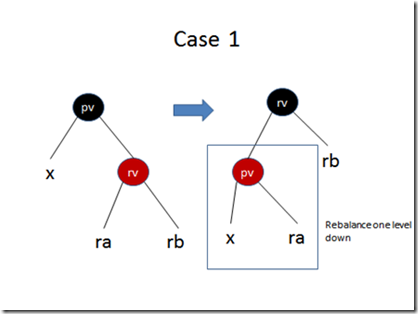(notations in the picture: nodes in circle are single nodes with colors, blue represents either red or black; a symbol represents a tree rooted at the symbol, for some cases the colors of the symbols are also noted.)

Case 2: If pv is of anycolor, and its right child is black. The three sub cases are shown below: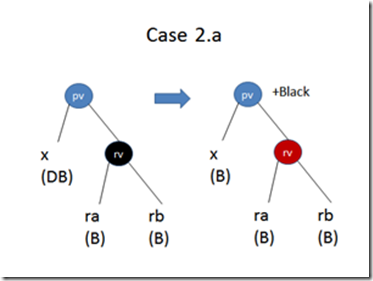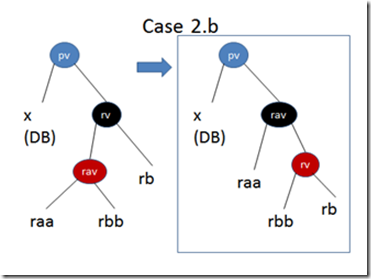And the balance procedure is as follows:

let rec balance = function
// invariant: the two children rooted at node pv have same "black" depth
// but the root node itself may become a "double" black node
| Black, pv, _, x, Node (Red, rv, size, ra, rb) when (isDoubleBlack x)-> // case 1
balance(Black, rv, 0, balance(Red, pv, 0, x, ra), rb) // do left-rotate and continue balance
| pc, pv, _, x, Node (Black, rv, size, ra, rb) when (isDoubleBlack x)-> // case 2.a 2.b and 2.c
if isBlack ra && isBlack rb then // 2.a: reduce a black on both side
let tempNode=Node (Red, rv, size, ra, rb)
Node (pc++Black, pv, (getSize x tempNode)+1, blackify x, tempNode) // reduces the double node to root
elif isBlack rb then // 2.b: do a right rotation in the right child, so rb becomes red and recudes to the "else" case
match ra with
| Node (_, rav, _, raa, rbb)->
let
tempNode1= Node (Red, rv, (getSize rbb rb)+1, rbb, rb)
let tempNode2=Node (Black, rav, (getSize raa tempNode1)+1, raa, tempNode1)
balance(pc, pv, 0, x, tempNode2)
| _->
failwith "impossible error"
else // 3.c
let tempNode=Node (Black, pv, (getSize x ra)+1, blackify x, ra)
Node (pc, rv, (getSize tempNode rb)+1, tempNode, blackify rb)

// when doubleblack x is on the right
| Black, pv, _, Node (Red, lv, _, la, lb), x when (isDoubleBlack x)->
balance(Black, lv, 0, la, balance(Red, pv, 0, lb, x))
| pc, pv, _, Node (Black, lv, size, la, lb), x when (isDoubleBlack x)->
if
isBlack la && isBlack lb then
let
tempNode=Node (Red, lv, (getSize la lb)+1, la, lb)
Node (pc++Black, pv, (getSize tempNode x)+1, tempNode, blackify x)
elif isBlack la then
match
lb with
| Node (_, _, lbv, lba, lbb)->
let
tempNode1=Node (Red, lv, (getSize la lb)+1, la, lba)
let tempNode2=Node (Black, lbv, (getSize tempNode1 lbb)+1, tempNode1, lbb)
balance(pc, pv, 0, tempNode2, x)
| _->
failwith "impossible error"
else
let
tempNode=Node (Black, pv, (getSize lb x)+1, lb, blackify x)
Node (pc, lv, (getSize la tempNode)+1, blackify la, tempNode)

| a, b, _, c, d->
Node (a, b, (getSize c d)+1, c, d)

Remember that this balance only balances the two children of any tree. Thus the root can still be DoubleDark (similar case in insert). We need a final check on the root:

match del x t with
| Node (_, y, size, a, b) ->
Node (Black, y, size, a, b)
| Leaf _ -> Leaf(Black)

Validation for the implementation

It is important to have some test to verify the correctness of the above implementation. My idea is to do some random insertions and deletions, and then after each insertion or deletion, check whether the tree still holds the three properties.

The validate function is given below:

let validate t =
let rec validate = function
| Leaf Black -> true, 0
| Leaf DoubleBlack ->
printfn "DoubleBlack in a Leaf node"
false, 0
| Leaf Red ->
printfn "Red in a Leaf node"
false, 0
| Node (Black, _, _, a, b) ->
let
lr, ln = validate a
let rr, rn = validate b
lr && rr && (ln = rn), ln + 1
| Node (Red, _, _, a, b) ->
match
a, b with
| Node (Red, _, _, _, _), _
| _, Node (Red, _, _, _, _) ->
let
lr, ln = validate a
printfn "Red-Red in a tree"
false, ln
| _ ->
let
lr, ln = validate a
let rr, rn = validate b
lr && rr && (ln = rn), ln
| Node (DoubleBlack, _, _, a, _) ->
let
lr, ln = validate a
printfn "DoubleBlack in an internal node"
false, ln
match t with
| Leaf Black -> true, 0
| Leaf _ -> false, 0
| Node (Black, _, _, _, _) ->
validate t
| Node (Red, _, _, _, _)
| Node (DoubleBlack, _, _, _, _) ->
printfn "root must be Black"
false, 0

I did use the above test case to catch one bug in the implementation, the original code for the final deletion step is:

match del x t with

| Node (_, y, size, a, b) ->

Node (Black, y, size, a, b)

| t -> t

This code has a bug that when the tree is deleted to empty, its representation is Leaf (DoubleBlack) rather than Leaf(Black)!

I did a performance test against with F#’s set, which uses AVL tree:

// Fisher-Yates shuffle
let randperm n =
let p = Array.init n (fun i -> i)
let r = new System.Random(1)
for i=n-1 downto 1 do
let
j = r.Next(i+1) // random number 0 <= j <= i
let tmp = p.[j]
p.[j] <- p.[i]
p.[i] <- tmp
p

let lst = randperm 1000000 |> Seq.toList

let rb = RBTree.ofList lst |> ignore

open Microsoft.FSharp.Collections

let av = Set.ofList lst |> ignore

//timing on a AMD E-350 Netbook (1.6 GHz)
// Real: 00:00:44.635, CPU: 00:00:44.694, GC gen0: 218, gen1: 61, gen2: 4
//
// val rb : unit = ()
//
// >
// Real: 00:00:17.448, CPU: 00:00:18.766, GC gen0: 76, gen1: 20, gen2: 2
//
// val av : unit = ()
//

As we can see, for a large set with one million elements, my implementation costs about two times of that of AVL tree in F# Core library. That was not bad. I shall devote more time into performance and see if we can do more improvement. One optimization is to reduce redundant tree node allocation.

Tree traversal

Let’s talk some interesting stuff about tree traversal at last. I did implement some traversal functions:

let rec map f = function
| Leaf _ as l -> l
| Node (color, x, size, l, r) -> Node (color, f x, size, map f l, map f r)

let rec iter f = function
| Leaf _ -> ()
| Node (color, x, size, l, r) ->
iter f l
f x
iter f r

let rec fold f t acc =
match t with
| Leaf _ -> acc
| Node (color, x, size, l, r) -> fold f l (f x (fold f r acc))

They are straightforward recursive functions, and not tail-recursive. For a balanced tree like red black tree, tail-recursive is not important because the tree does not go too deep (a tree with 1 to 1000000 in it has a depth of 26). But it is just fun to make tem tail-recursive by using continuations. The standard accumulating variable trick does not work here because we have two recursive calls.

Let’s write the tail-recursive form of map function:

let map2 f t =
let rec map f t k =
match t with
| Leaf _ as l -> k l
| Node (color, x, size, l, r) ->
map f l (fun lmap ->
map f r (fun rmap -> k (Node(color, f x, size, lmap, rmap))))

map f t (fun x -> x)

We can also use a Monad to abstract the continuation pattern out:

type Cont<'a,'r> = ('a -> 'r) -> 'r

type ContBuilder() =
member x.Return (a):Cont<'a,'r> = fun k -> k a
member x.Bind (c:Cont<'a,'r>, f:'a -> Cont<'b,_>) =
(fun k -> c (fun a -> f a k))
member this.Delay(f) = f()

let cont = ContBuilder()

let map3 f t =
let rec map f = function
| Leaf _ as t -> cont { return t }
| Node (color, x, size, l, r) ->
cont {
let! lm = map f l
let! rm = map f r
return Node (color, f x, size, lm, rm)
}
map f t (fun x -> x)

A note on <'a when 'a:comparison>

In our definition of the Red Black Tree:

type RBTree<'a when 'a:comparison> =
| Node of Color * 'a * int * RBTree<'a> * RBTree<'a>
| Leaf of Color

The type signature has an ad hoc construct: when ‘a: comparison. Actually the only other constraint on ‘a is equality. F# only supports these two type constraints.

Type constraints behave like type classes in Haskell. :equality is Eq, :comparison is Ord. However, type classes in Haskell are much flexible.

Details could be found on this blog article by Don Syme.

Summary

In this article, I have implemented a functional Red Black Tree with dynamic order statistics. The tree is complete and has been well tested. Before any performance tuning, the running speed of it is within a small factor (2-3) of the AVL tree in F# Core library.

This article shows many important features of functional programming: 1) pattern matching and algebraic data types help implement complex data structures; 2) recursion is everywhere and the correctness of the program could be proved by inductive reasoning; and finally 3) a light introduction to tree traversal and continuation and Monad in F#.

Sunday, August 28, 2011

N-queen problem, F#, Scheme and Haskell!

I am busy with my research and other F# work, I haven’t written blog articles recently. But I always keep an eye on interesting things on F#. The F# version of “Write Yourself a Scheme in 48 hours” by Loca Bologonese really caught my eyes. Luca took the Haskell version and translated it into F# with the main structure remained the same. This gives me (an F# programmer) a great opportunity to learn Haskell by reading the F# and the Haskell programs side by side! It is really a good learning experience (previously I know a little Haskell).

N-Queen in F# and Scheme

The fun part was that I wished to write a functional version of n-queen program in Scheme and see the execution speeds of the F# evaluator and the Haskell evaluator!

n-queen problem is one of my favorite puzzles. I learned depth first search/backtracking with this problem. It is a good exercise for how to write a non-trivial recursive program other than factorial or Fibonacci sequence.

As the readers of this blog are probably F# users, I gave an F# version first in case you are not familiar with Scheme. The scheme version of the program uses exactly the same idea and same data structures.

The F# version:

// check if the two queens attack each other
let conflict (i1, j1) (i2, j2) =
if i1 = i2 || j1 = j2 || abs(i1-i2) = abs(j1-j2) then
true
else
false

// check if a new position is valid to a list of other (valid) positions
let check (newPos:int*int) (posList: (int*int) list) =
posList
|> List.exists (fun pos -> conflict newPos pos)
|> not

// the backtracking procedure to get all solutions
let rec searchQueen i n sols =
if i = n then
sols
else
let
newSols =
sols
|> List.map (fun sol ->
[0..n-1]
|> List.filter (fun j -> check (i,j) sol)
|> List.map (fun j -> (i,j)::sol)
)
|> List.concat
searchQueen (i+1) n newSols

let allSolutions = searchQueen 0 8 [[]]

allSolutions |> List.length

The scheme version:

;; helper functions
(define (accumulate op init seq)
(if (null? seq)
init
(op (car seq)
(accumulate op init (cdr seq)))))

(define (accumulate op init seq) (if (null? seq) init (op (car seq) (accumulate op init (cdr seq)))))

(define (flatmap proc seq)
(accumulate append '() (map proc seq)))

(define (enumerate-interval low high)
(if (> low high)
'()
(cons low (enumerate-interval (+ low 1) high))))

;; data structures and basic functions for board
(define (make-queen row col) (list row col))
(define (get-row queen) (car queen))
(define (get-col queen) (car (cdr queen)))

(define (same-col? q1 q2) (= (get-col q1) (get-col q2)))
(define (same-diag? q1 q2)
(=
(abs (- (get-row q1) (get-row q2)))
(abs (- (get-col q1) (get-col q2)))))

(define (attacks? q1 q2)
(or (same-col? q1 q2) (same-diag? q1 q2)))

(define (safe? newq qlist)
(cond
((null? qlist) #t)
((attacks? newq (car qlist)) #f)
(else (safe? newq (cdr qlist)))))

(define (safe-board? qlist)
(let
((newq (car qlist))
(rest (cdr qlist)))
(safe? newq rest)))

;; the depth-first search for queens
(define (queens board-size)
(define (queen-rows k sols)
(if (= k board-size)
sols
(queen-rows (+ k 1)
(filter
(lambda (board) (safe-board? board))
(flatmap
(lambda (rest-queens)
(map (lambda (new-col)
(cons (list k new-col) rest-queens))
(enumerate-interval 1 board-size)))
sols)))))
(queen-rows 0 (list '())))

(length (queens 8))

Here are some correspondences between the F# version and the scheme version:

1. In F#, each queen position is represented as a tuple (int * int), while in Scheme, each queen position is a list with length 2.

2. In the search procedure, the main logic is: given a list of sub-solutions (sols), expand every one with all possible columns for the new position. This gives a list of lists of lists of positions. There should be a flatten function to transform it into a list of lists of positions. In F#, it is List.concat, in Scheme, it is flatmap.

Run n-queen in the Scheme interpreters

The fun part is to run the n-queen program in the F# version interpreter. However, throwing the above scheme program into the interpreter gives errors. (It runs well in CzScheme)

Here are the limitations that I encountered:

1. The interpreter only supports one-line definitions. Overcoming this limitation is easy – just write the functions in one-lines.

2. The interpreter does not support (let). let syntax gives a word for some expression. The easy way is to use the expression directly.

3. Some built-in functions are not included in the interpreter, e.g. abs and append. Implementing them is quite easy.

4. The interpreter uses Console.ReadLine() to read from the input, which only supports 256 characters per line by default. The following lines reset the buffer size to allow more characters per line:

let inputBuffer = Array.create 1024 0y          let inputStream = Console.OpenStandardInput(inputBuffer.Length)         Console.SetIn(new IO.StreamReader(inputStream))

5. Does not support nested-define. Nested-define could be implemented as let + lambda. But I didn’t solve this problem in this post. I just remove nested-defines.

Here is the runnable Scheme program:

(define (abs x) (if (negative? x) (- 0 x) x))
(define (append list1 list2) (if (null? list1) list2 (cons (car list1) (append (cdr list1) list2))))
(define (accumulate op init seq) (if (null? seq) init (op (car seq) (accumulate op init (cdr seq)))))
(define (flatmap proc seq) (accumulate append (quote ()) (map proc seq)))
(define (enumerate-interval low high) (if (> low high)    '()      (cons low (enumerate-interval (+ low 1) high))))
(define (make-queen row col) (list row col))
(define (get-row queen) (car queen))
(define (get-col queen) (car (cdr queen)))
(define (same-col? q1 q2) (= (get-col q1) (get-col q2)))
(define (same-diag? q1 q2)   (=    (abs (- (get-row q1) (get-row q2)))    (abs (- (get-col q1) (get-col q2)))))
(define (attacks? q1 q2)  (or (same-col? q1 q2) (same-diag? q1 q2)))
(define (safe? newq qlist)  (if     (null? qlist) #t    (if (attacks? newq (car qlist)) #f  (safe? newq (cdr qlist)))))
(define (safe-board? qlist)  (safe? (car qlist) (cdr qlist)))
(define board-size 7)
(define (queen-rows k sols) (if (= k board-size)   sols (queen-rows (+ k 1)           (filter             (lambda (board) (safe-board? board))            (flatmap        (lambda (rest-queens)        (map (lambda (new-col)      (cons (list k new-col) rest-queens))       (enumerate-interval 1 board-size)))       sols)))))
(length (queen-rows 0 (list (quote ()))))

The above program runs OK in both F# and Haskell interpreter at about the same speed. However, when board-size equals 8, F# version gets a Stackoverflow runtime error.

I think it is enough for a post, I will see if I can solve these problemsMonday, April 18, 2011

Extracting top words from titles and abstractions in MIX11 presentations

The videos of MIX11 (Apr. 11-14) conference are available at http://channel9.msdn.com/Events/MIX/MIX11. To get a sense of what’s going on recently, I wrote an F# script to count the top occurring words in the titles and abstractions of all the presentations.

Title statistics:

"windows" : 41
"phone" : 26
"azure" : 14

"web" : 14
"html5" : 11
"silverlight" : 9

"net" : 9
"7" : 8
"applications" : 8
"data" : 8
"platform" : 7
"application" : 7
"javascript" : 7
"new" : 7
"ux" : 6
"what’s" : 6
"asp" : 6
"boot" : 5
"camp" : 5
"building" : 5

From the statistics of the words in titles, we can find that windows mobile phone and azure cloud platform are the hottest topics.  HTML5, the next standard of web page technology, has been always been a focus by Microsoft. Silverlight still has its heat. It is a good company to HTML5, I think it will have many applications in in-house web applications; while HTML5 has more support and available in all browsers across platforms.

Abstract statistics:

"web" : 62
"session" : 55
"windows" : 49
"new" : 40
"applications" : 36
"phone" : 36
"learn" : 32
"we’ll" : 29
"use" : 25
"using" : 24
"silverlight" : 23
"data" : 23
"net" : 22
"javascript" : 21
"azure" : 21
"developers" : 20
"come" : 17
"microsoft" : 17
"features" : 17
"one" : 16

and the whole F# program is actually short -- only 40 lines! You have everything there: download webpages, get the titles and titles, stopword removing and word counting, sorting...

Code Snippet
1. open System
2. open System.Net
3. open System.Text.RegularExpressions
4.
5. let fetchUrlSimple (url:string) =
6.     let req = WebRequest.Create(url)
7.     let response = req.GetResponse()
8.     use stream = response.GetResponseStream()
11.
12. let topKWords (docs:string seq) K =
13.     let separator = [|' '; '\r'; '\n'; '-'; '.'; ',' ; '\t'; '!'; '?'; '\''; ';'; '/' |]
15.
16.     docs
17.     |> Seq.map (fun doc ->
18.         doc.Split(separator, StringSplitOptions.RemoveEmptyEntries)
19.         |> Seq.map (fun word -> word.ToLower())
20.         |> Seq.filter (fun word -> not (stopwords.Contains(word)))
21.         )
22.     |> Seq.concat
23.     |> Seq.groupBy (fun x->x)
24.     |> Seq.map (fun (word, wordSeq) -> (word, wordSeq |> Seq.length))
25.     |> Seq.sortBy (fun (_, wordCnt) -> - wordCnt)
26.     |> Seq.take K
27.     |> Seq.toList
28.
29. let rawpage = fetchUrlSimple @"http://channel9.msdn.com/Events/MIX/MIX11"
30. let titles, abstracts =
31.     let page = Regex.Replace(rawpage, "&#?[a-z0-9]+;", " ")
32.     let titleMatches = Regex.Matches(page, "class=\"title\">(.*?)</a>")
33.     let abstractMatches = Regex.Matches(page.Replace("\n"," "), "class=\"description\">(.*?)</div>")
34.     let matchesToSeq (matches: MatchCollection) =
35.         seq {
36.             for m in matches do
37.                 yield m.Groups..Value
38.         }
39.     matchesToSeq titleMatches, matchesToSeq abstractMatches
40.
41.
42. topKWords titles 20
43. topKWords abstracts 20

My colleague Defu Lian wrote a C# version for topKWords function using LINQ:

Code Snippet
1. static List<Tuple<string, int>> topKWords(IEnumerable<string> docs,int K)
2. {
3.     char[] separator = { ' ', '\r', '\n', '-', '.', ',', '\t', '!', '?', '\'', ';', '/' };
5.     return docs.SelectMany(doc => doc.Split(separator, StringSplitOptions.RemoveEmptyEntries)
6.                         .Select(word => word.ToLower())
7.                         .Where(word => !stopwords.Contains(word)))
8.         .GroupBy(a => a)
9.         .Select(wordgroup => Tuple.Create<string,int>(wordgroup.Key, wordgroup.Count()))
10.         .OrderByDescending(w2c => w2c.Item2)
11.         .Take(K)
12.         .ToList();
13. }

Sunday, April 10, 2011

A Note on F# Quotations

I worked on extending the F# ODSL (Optimization Domain Specific Language, link1 and link2) during the weekend. This is the first time I use F# quotations in a non-trivial fashion. I tried some quotation examples in Programming F# when I was learning the language and read code examples in F# PowerPack (for LINQ integration) and other libraries before.

Only after I actually write some programs in it, I can appreciate this F# feature more and have some of my own thoughts. In this post, I’d like to share these thoughts. I don’t intend to go into detailed F# code, but maybe in the future I will write a separate blog on my work on extending the F# ODSL.

General idea of F# Quotations

The great idea of quotation at least traces back to Lisp, where program is also a kind of data – the execution behavior of a piece of program is completely controllable by the user, just treat it as input data and write a custom evaluator for it. The default Lisp evaluator is eval, we can easily write a custom one to change the default behavior [LispEval].

In F#, we can also treat a piece of F# code as data by quoting it:

let quotedProgramAsData =
<@
let y = 1 + 2 * 30
20 * y
// code here:
// an F# program using a subset of F# language features
@>

When we create the variable quotedProgramAsData, the .Net runtime will not compute the expression in the quotation; instead it generates the following value:

val quotedProgramAsData : Quotations.Expr<int> =

val quotedProgramAsData : Quotations.Expr<int> =
Let (y,
[Value (1),
Call (None, Int32 op_Multiply[Int32,Int32,Int32](Int32, Int32),
[Value (2), Value (30)])]),
Call (None, Int32 op_Multiply[Int32,Int32,Int32](Int32, Int32),
[Value (20), y]))

Let’s visualize it in a tree form:

F# compiler generates this tree structure by free and the rest is how you deal/evaluate this tree. If the above code is not in quotation, F# compiler will generate code that put the value of the second parameter (1 + 2 * 30) of Let to the first parameter (y), and continue to generate code for the third parameter (20 * y). But because it is in the quotation, only the expression tree is generated without any explicit execution behavior for them.

With different purposes, we can write different evaluators for the quoted F# code. In the following I list some of the application areas.

Domain specific language (DSL)

There are different kinds of domain specific languages. In his book Domain Specific Languages, Martin Fowler summarizes them into external and internal ones:

DSLs come in two main forms: external and internal. An external DSL is a language that's parsed independently of the host general purpose language: good examples include regular expressions and CSS. External DSLs have a strong tradition in the Unix community. Internal DSLs are a particular form of API in a host general purpose language, often referred to as a fluent interface. The way mocking libraries, such as JMock, define expectations for tests are good examples of this, as are many of the mechanisms used by Ruby on Rails. Internal DSLs also have a long tradition of usage, particularly in the Lisp community. (http://martinfowler.com/books.html#dsl)

Quotations can be used as a very good language feature for implementing internal domain specific languages.

As stated as before, the quoted program is anyway an F# program. How could it be look like a domain specific one? Remember F# has a rich set of syntax while a domain language takes a small subset of it is usually enough expressive.

Take a look at the following program, which expresses the quadratic optimization for Support Vector Machines:

// solve the SVM using ODSL
let dsl_solver =
let index = [ 0..n-1]
Solver
<@
qp()
let alpha = vararray1 (index)
maximise (sum index (fun i -> alpha.[i]) -
(sum index (fun i -> sum index (fun j ->
coef.[i,j] * alpha.[i] * alpha.[j])))) // Eq. (1)
where
[
foreach index (fun i -> 0.0 <= alpha.[i]) // Eq. (2)
foreach index (fun i -> alpha.[i] <= C) // Eq. (2)
sum index (fun i -> alpha.[i] * y.[i]) = 0. // Eq. (3)
]
@>

and its optimization formulation:

Subject to constraints:

The correspondence between the DSL code and mathematical formulates is very clear. In the future work, we can even vectorize this DSL to eliminate the usage of sum: range -> lambda (int->value) -> value function, which will make the program and the formulas more similar.

Note that in the above example qp, maximise, sum and where are not magic, but are all F# functions whose behaviors are defined in the DSL. For example, F# function qp() only indicates the following code is a quadratic programming.

Compare this code with the code in my SVM post: it is clearer and thus easier to write.

The restriction of such a DSL inside F# is that the syntax of this DSL should follow that of F# -- it should be a valid program recognizable by the F# lexer and parser. This is why DSL people like Lisp which has so simple and flexible syntax (just brackets), but sometimes it is also boring and confusing when one gets lost in the brackets.

High performance computing

We know that F#’s Seq module and C#’s LINQ share some common features, e.g. chained operations and the laziness. Because these operations are so fundamental, LINQ team has spent enormous time optimizing LINQ; on the other hand, F#’s implementation is quite a standard one without heavy optimization because F# compiler team is not big and they have other important tasks to do. (F#’s Seq module actually does some optimization, e.g. for arrays and lists special routines are called instead of the general ones for IEnumerable<T> objects. But LINQ does more!)

So one idea of speeding up sequence operations in F# is to use LINQ’s equivalent functions. This could be implemented by putting F#’s sequence operations in a quotation and rewrite them in LINQ expression as done in F# PowerPack. Interested readers can read the source code of LINQ for F# in PowerPack.

The above example talks about basic data structures. As a data mining guy myself, let’s move to numerical computing: we can quote a piece of numerical code in F# and translates it into Fortran/C and a JIT Fortran/C compiler compiles the code translated code into native machine code which uses special instruction set in that platform (e.g. vectorized instructions in P4 CPU). Or even we can compile this piece of F# into GPU and utilize the parallel computing there. [Syme_ML06]

But any fancy optimization has some overhead too. For example, the compiling time may cost some time.

Education: Compiler courses

The last application area is education. There are few CS departments teaching functional programming. Some of them will mention it when teaching programming language concepts. F# is a push for FP into industry and main stream. Hope in the near future, schools will open tiny or selected courses for F#. See the recent F# in Education Workshop [Education] for details.

Ok. Let’s focus on compiler courses. Compilers, different from functional programming, are taught in nearly all CS departments. However the projects in compiler courses are a huge pain to students. They are so complicated! And the focus of the first half of the course (lexing and parsing) and the second half (code generation and optimization) are kind of separate. The theories behind the two halves are different. Sometimes, the course instructor focuses too more on lexing and parsing and don’t have enough time for students to work on code generation and optimization, which in my mind are more important in a compiler course. While lexing and parsing will occur in other CS courses too, e.g. computational theory, code generation and optimization will only be in a compiler course.

By using the F# quotations, F# compiler generates the expression tree for free. Just so convenient and straightforward! Considering that the F# syntax you can put in quotation is a capable set of imperative language + functional language, the expression tree for us to do the code generation and optimization is non-trivial. By using tools like .Net Reflector, we can also study how the standard F# compiler generates the same piece of code and learn the tricks there. Students can work on code generation for closures, and tail-recursive-calls – important language features that are not implemented or fully implemented in many main stream languages.

References

Some references in the above text:

[LispEval] Christian Queinnec , Lisp in Small Pieces.

[Syme_ML06] Leveraging .NET Meta-programming Components from F#, ML Workshop, 2006.

[Education] F# in Education Workshop, http://research.microsoft.com/en-us/events/fsharpined/.

A very good paper by Leijen and Meijer:

[Leijen&Meijer] Domain specific embedded compilers, Sigplan Notices, vol. 35, no. 1, pp. 109-122, 2000.

F# quotation extensively uses active patterns for expression tree pattern matching:

[Syme_Active] Extensible Pattern Matching via a Lightweight Language Extension, ICFP 2007.

Here are some relevant blog posts:

[Petricek] F# Overview (IV.) - Language Oriented Programming, http://tomasp.net/blog/fsharp-iv-lang.aspx.

[RubyDSL] Building a DSL in Ruby, http://jroller.com/rolsen/entry/building_a_dsl_in_ruby.

Two nice answers by Petricek & Harrop discussing Quotations (F#, Ocaml and Lisp) and DSL on Stackoverflow:

Acknowledgement

I’d like to thank Nathan Brixius for discussions on F# ODSL!

Thursday, February 24, 2011

Support vector machines (SVMs) in F# using Microsoft Solver Foundation

Support vector machines are a super star in machine learning and data mining in the past decade. It reheats statistical learning in machine learning community. It is also one of the classifiers that work practically with applications to other disciplines such as bioinformatics.

In this post, I will give a brief review of the theory of this classifier and show an F# implementation using the quadratic programming (QP) solver in Microsoft Solver Foundation (MSF).

It may seem dull to start with mathematics. But the math in SVMs is actually quite intuitive and would be quite easy if one has some training in linear algebra and calculus. Also understanding the intuition behind the formulae is more important in the formulation itself. There are quite a lot of good SVM tutorials written by researchers. Thus I only write the important formulas that occur in the implementation. The reader could link the F# code with formulas directly.

My note is based on the machine learning course by Prof. Jaakkola [Jaakkola] and the Matlab implementation therein. The course material is highly comprehensive yet accurate.

Background review for SVMs

Given a binary labeled dataset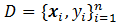, whereandis 1 or -1. Think about the data points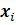plotted in a d dimensional (Euclidean) space, the linear SVM classifier is a hyperplane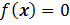in the space and it “best” separates the two kinds of data points. Here “best” means that the hyperplane should have the largest margin, which is the distance from the plane to the sides of labeled points. Intuitively the larger the margin is, the more robust and confident the classifier is. As if the hyperplane shakes a little, it still classifies well for its being far from both sides.

Let’s take d=2 as the discussing example: data points are plotted in a 2-D plane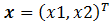. The aim is to draw a line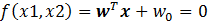to separate the two kinds of points such that the margin of the separation is maximized. In the following Figure, the line with maximum margin is shown. After some geometry work, the margin is calculated as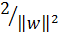, thus minimizing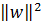with the constraints that the two kinds of points are well separated (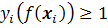) gives the max-margin hyperplane.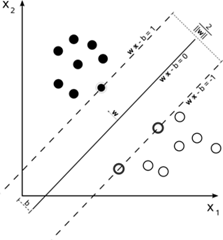(Wikipedia picture)

Two important extensions to the above simplest SVM are:

1. Allowing imperfect separation, that is when a hyperplane could not separate the points perfectly, it is allowed to mis-separate some data points. This introduces a slack variable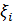for each data point, when the point is on the correct side of the plane, the slack variable is 0, otherwise it is measures the distance it goes from the plane minus 1, if the values goes to below zero set it zero. (Please read the Note and reference section for more explanation.)

2. Kernel. The simplest SVM is a linear classifier in the original space. However, there are situations where linear classifier is not sufficient (even the best), one strategy is to do a non-linear transformation or mapping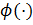that maps a data point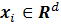to another space, so that a linear classifier in that space, which is non-linear in the original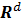space, does a better separation of the data points. The kernel trick is that we don’t need to defineexplicitly; only the definition of inner product of that space is required: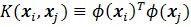.

With the two extensions, the new maximum margin objective becomes: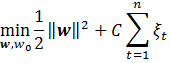subject to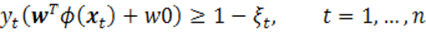The dual form: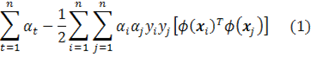subject to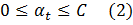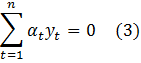The data points with its alpha value greater than 0 are called support vectors.

With the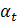in the dual problem solved, the SVM classification hyperplane is recovered by: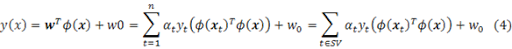The threshold parameter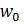could be calculated by substituting back to the support vectors: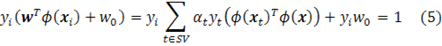Any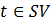and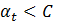(So that make sure data pointis on the margin boundary, not in-between) would give a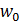, to stabilize the solution, it is common practice to take the median of off the possible.

The implementation

The solver is actually an Interior Point Solver, which could solve linear and quadratic programming with linear constraints.

Page 14-17 of MSF-SolverProgrammingPrimer.pdf show an example usage of the QP solver in Microsoft Solver Foundation. But the example is not complete and it does not demonstrate one very subtle part: how to set the coefficients for the quadratic terms.

The following code example shows a simple example with the the comment regarding the coefficient setting:

Example usage of the QP solver
1. #r @"C:\Program Files (x86)\Sho 2.0 for .NET 4\packages\Optimizer\Microsoft.Solver.Foundation.dll"
2. open Microsoft.SolverFoundation.Common
3. open Microsoft.SolverFoundation.Solvers
4. open Microsoft.SolverFoundation.Services
5. // test QP
6. module TEST =
7. (* minimize x^2 + y^2 + 3xy + 2x + y *)
8. (* notice that in the InteriorPointSolver,
9. the coefficients for xy & yx should be the same (so only set ONCE!)
10. if we set both 3xy and 0yx, the solver takes the later coef.
11. *)
12. let solver = new InteriorPointSolver()
13. let _, goal = solver.AddRow("dual objective value")
15. let _, x = solver.AddVariable("x")
16. let _, y = solver.AddVariable("y")
17. solver.SetCoefficient(goal, x, Rational.op_Implicit(2))
18. solver.SetCoefficient(goal, y, Rational.op_Implicit(1))
19. // for terms like x-y (where x != y), set its coef for only one time!
20. solver.SetCoefficient(goal, Rational.op_Implicit(3), x, y)
21. //solver.SetCoefficient(goal, Rational.Zero, y, x)
22. solver.SetCoefficient(goal, Rational.op_Implicit(1), x, x)
23. solver.SetCoefficient(goal, Rational.op_Implicit(1), y, y)
24. let param = new InteriorPointSolverParams()
25. solver.Solve(param) |> ignore
26. //solver.Result
27. let objectiveValue = solver.GetValue(0).ToDouble()
28. let x0 = solver.GetValue(1).ToDouble()
29. let y0 = solver.GetValue(2).ToDouble()
30. x0*x0 + y0*y0 + 3.0 * x0 * y0 + 2. * x0 + y0
31. //x0*x0 + y0*y0 + 0.0 * x0 * y0 + 2. * x0 + y0

The implementation of SVM is a straightforward translation of equations (1) to (5). The following shows the svm learning (bulidSvm) and testing (svmClassify) functions:

SVM Implementation
1. open System
2. type dataset =
3. { features: float array array; // (instance = float array) array
4. mutable labels: int array; //
5. }
6. with
7. member x.NumSamples = x.features.Length
8. module Array =
9. let median (a:'a array) =
10. let sorted = Array.sort a
11. sorted.[sorted.Length / 2]
12. module Kernel =
13. let linear a b =
14. Array.fold2 (fun acc p q -> acc + p * q) 0.0 a b
15. let polynomial k a b =
16. let dot = linear a b
17. Math.Pow(1.0 + dot, k |> float)
18. let gaussian beta a b =
19. let diff = Array.fold2 (fun acc p q -> acc + (p-q)*(p-q)) 0.0 a b
20. exp (-0.5 * beta * diff)
21. module SVM =
22. type svmmodel = {
23. SVs:dataset;
24. alpha:float array;
25. kernel: float[] -> float[] -> float;
26. w0:float;
27. }
28. with
29. member x.NumSupporVectors = x.SVs.features.Length
30. let buildSVM (ds:dataset) (C:float) (kernel:float[] -> float[] -> float) =
31. let n = ds.features.Length
32. let C = Rational.op_Implicit(C)
33. let zero = Rational.Zero
34. // create a interior point solver, which solves the QP problem
35. let solver = new InteriorPointSolver()
36. // set the objective value / goal
37. let _, goal = solver.AddRow("dual objective value")
38. // false == maximizing the objective value
39. // the value of goal is (1)
40. solver.AddGoal(goal, 0, false) |> ignore
41. // add the Lagangian variables \alpha_i and set their bounds (0 <= \alpha_i <= C)
42. let alpha = Array.create n 0
43. for i=0 to n-1 do
44. let _, out = solver.AddVariable("alpha_"+i.ToString())
45. alpha.[i] <- out
46. solver.SetBounds(out, zero, C)
47. // add contraint: \sum_i \alpha_i * y_i = 0
48. // equation (2)
49. let _, sumConstraint = solver.AddRow("SumConstraint")
50. solver.SetBounds(sumConstraint, zero, zero);
51. for i=0 to n-1 do
52. // set the coefs for the sum constraint
53. // equation (2)
54. solver.SetCoefficient(sumConstraint, alpha.[i], Rational.op_Implicit(ds.labels.[i]))
55. // add the \alpha_i terms into the objective
56. solver.SetCoefficient(goal, alpha.[i], Rational.One)
57. // add the qudratic terms
58. for j=0 to i do
59. // coef = y_i * y_j * K(x_i, x_j)
60. let coef = float(ds.labels.[i] * ds.labels.[j]) * (kernel ds.features.[i] ds.features.[j])
61. if i=j then
62. solver.SetCoefficient(goal, Rational.op_Implicit(-0.5 * coef), alpha.[i], alpha.[j])
63. else
64. solver.SetCoefficient(goal, Rational.op_Implicit(-coef), alpha.[i], alpha.[j])
65. // use the default parameters
66. let param = new InteriorPointSolverParams()
67. solver.Solve(param) |> ignore
68. // get the alpha values out
69. let alphaValue = Array.init n (fun i -> solver.GetValue(i+1))
70. (* print optimization result
71. printfn "goal value = %A" (solver.GetValue(0).ToDouble())
72. for i=1 to n do
73. printfn "%A" (solver.GetValue(i).ToDouble())
74. *)
75. let alphaNew = new ResizeArray<Rational>()
76. // extract the non-zero alpha values out and their corresponding support vectors
77. let SVs =
78. let feats = new ResizeArray<float[]>()
79. let labs = new ResizeArray<int>()
80. let maxAlpha = Array.max alphaValue
81. let threshold = maxAlpha * Rational.op_Implicit(1e-8)
82. for i=0 to n-1 do
83. if alphaValue.[i] > threshold then
87. { features = feats |> Seq.toArray;
88. labels = labs |> Seq.toArray;
89. }
90. // solve w_0 in the primal form
91. let alphaNZ = alphaNew |> Seq.toArray
92. // equation (5)
93. let w0 =
94. alphaNZ
95. |> Array.mapi (fun i a ->
96. if a = C then
97. None
98. else
99. let mutable tmp = 0.0
100. for j=0 to SVs.NumSamples-1 do
101. tmp <- tmp + alphaNZ.[j].ToDouble() * (SVs.labels.[j] |> float) * (kernel SVs.features.[i] SVs.features.[j])
102. Some ((float SVs.labels.[i]) - tmp)
103. )
104. |> Array.filter (fun v -> match v with None -> false | _ -> true)
105. |> Array.map (fun v -> match v with Some v -> v | _ -> 0.0)
106. |> Array.median
107. // construct an svm record
108. {
109. SVs = SVs;
110. alpha = alphaNZ |> Array.map (fun v -> v.ToDouble());
111. kernel = kernel;
112. w0 = w0;
113. }
114. let svmClassify (model:svmmodel) (ds:dataset) =
115. // equation (4)
116. let vals =
117. ds.features
118. |> Array.map (fun x ->
119. let mutable sum = 0.0
120. for i=0 to model.NumSupporVectors-1 do
121. sum <- sum + model.alpha.[i] * (float model.SVs.labels.[i]) * (model.kernel model.SVs.features.[i] x)
122. sum + model.w0
123. )
124. let nCorrect =
125. Array.map2 (fun value label -> if (value > 0.0) && (label = 1) || (value < 0.0) && (label = -1) then 1 else 0) vals ds.labels
126. |> Array.sum
127. (float nCorrect) / (float ds.NumSamples), vals

Try on the iris data set we used in the Logistic Regression post:

let svm = buildSVM iris 10.0 Kernel.linear
let classifyResult = svmClassify svm iris

val svm : svm =
{SVs =
{features =
[|[|1.0; 6.2; 2.2; 4.5; 1.5|]; [|1.0; 5.9; 3.2; 4.8; 1.8|];
[|1.0; 6.3; 2.5; 4.9; 1.5|]; [|1.0; 6.7; 3.0; 5.0; 1.7|];
[|1.0; 6.0; 2.7; 5.1; 1.6|]; [|1.0; 5.4; 3.0; 4.5; 1.5|];
[|1.0; 4.9; 2.5; 4.5; 1.7|]; [|1.0; 6.0; 2.2; 5.0; 1.5|];
[|1.0; 6.3; 2.7; 4.9; 1.8|]; [|1.0; 6.2; 2.8; 4.8; 1.8|];
[|1.0; 6.1; 3.0; 4.9; 1.8|]; [|1.0; 6.3; 2.8; 5.1; 1.5|];
[|1.0; 6.0; 3.0; 4.8; 1.8|]|];
labels = [|-1; -1; -1; -1; -1; -1; 1; 1; 1; 1; 1; 1; 1|];};
alpha =
[|6.72796421; 10.0; 10.0; 10.0; 10.0; 6.475497298; 1.490719712;
8.547262902; 3.165478894; 10.0; 10.0; 10.0; 10.0|];
kernel = <fun:svm@226-32>;
w0 = -13.63716815;}

>
Real: 00:00:00.002, CPU: 00:00:00.000, GC gen0: 0, gen1: 0, gen2: 0

val classifyResult : float * float [] =
(0.97,
[|-2.787610618; -2.380530973; -1.42477876; -2.929203541; -1.681415929;
-1.96460177; -1.24778761; -6.106194692; -2.761061946; -2.973451328;
…. 4.203539824; 1.82300885|])

Notes and references

The optimization in SVMs could be treated as a general QP problem as shown in our implementation. However, when the number of the data points is big, the QP grows too big to handle by the QP solver.

As a special QP problem, SVM has a lot of novel solutions proposed in the machine learning research area, e.g. the SMO approach [Platt] in LibSVM implementation and the Ball Vector Machine approach [Tsang] transforming the special QP into a (discrete) computational geometry problem, minimum enclosing ball. For practical usage of SVM, one usually uses these dedicated approaches.

Besides the maximum margin interpretation, SVMs also have theory roots in functional regularization theory, in which the optimizationis viewed as minimizing the error term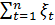while controlling the complexity of the function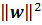and C is a tradeoff parameter between these two. The reader is referred to the teaching notes and papers by Prof. Poggio [Poggio].

[Burges] A Tutorial on Support Vector Machines for Pattern Recognition. http://research.microsoft.com/en-us/um/people/cburges/papers/svmtutorial.pdf

[Jaakkola] http://courses.csail.mit.edu/6.867/.

[Platt] Sequential Minimal Optimization: A Fast Algorithm for Training Support Vector Machines. ftp://ftp.research.microsoft.com/pub/tr/tr-98-14.pdf

[Poggio] http://www.mit.edu/~9.520/spring10/.